# AP Microeconomics : Perfectly Competitive Labor Markets

## Example Questions

### Example Question #1 : Competition

The following question will refer to this table: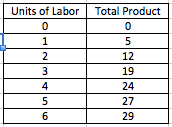What is the marginal product of the fourth unit of labor?

Possible Answers:

None of the other answers

5

12

19

24

Correct answer:

5

Explanation:

The marginal product of labor is the change in output that results from an additional unit of labor. In this case, the marginal product of the fourth unit of labor would be the difference between the total output of production with four units of labor and the total output of production with three units of labor, which is 5 units.

### Example Question #1 : Perfectly Competitive Markets

A minimum wage for coal miners will likely result in:

Possible Answers:

A shortage of coal miners

An increase in the demand for coal miners

A decrease in the supply of coal miners

A surplus of coal miners

Lower wages for coal miners

Correct answer:

A surplus of coal miners

Explanation:

Imposing a minimum wage usually causes a price floor in the market. Thus, the labor market for coal miners will likely see a surplus, which typically occurs when there is a price floor. The price will be higher than the equilibrium, which means that the labor supply will be greater than demand.

### Example Question #3 : Competition

The following question is based on this table: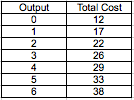What is the total variable cost of producing five goods?

Possible Answers: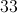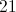Cannot be determined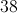Correct answer:Explanation:

Variable costs associated with production are costs that change in proportion to the level of production. By contrast, fixed costs are costs that are not dependent on production level. Fixed costs and variable costs make up a good's total cost of production.

In this case, the total cost of producing zero units is 12. The total variable cost of producing zero units is 0, which means that 12 must be the fixed cost associated with producing this good.

The total cost of producing 5 units is 33. Since total costs subtracted by fixed costs equals its variable costs, the variable cost of producing 5 units must be 21.

### Example Question #2 : Competition

If the total product of labor increases at an increasing rate, _________ .

Possible Answers:

marginal product of labor is at its maximum.

marginal product of labor is constant.

marginal product of labor is increasing.

marginal product of labor is decreasing.

marginal product of labor is at its minimum.

Correct answer:

marginal product of labor is increasing.

Explanation:

Marginal product of labor describes how the total product of labor changes when more labor is hired. If the total product of labor is rising as labor increases, then the marginal product of labor must be increasing.

### Example Question #1 : Perfectly Competitive Labor Markets

A perfectly competitive firm produces at its profit maximizing level. At this point, the marginal product of labor is 7 units per hour and wages are $14 per hour. Which of the following is true? Possible Answers: The market price of the good is$3 per unit.

The cost of producing the good is $3 per unit. The market price of the good is$2 per unit.

None of the other answers are correct.

The cost of producing the good is $2 per unit. Correct answer: The market price of the good is$2 per unit.

Explanation:

To maximize profits, a perfectly competitive firm will hire until its marginal revenue of labor equals its marginal cost of labor. The marginal cost of labor equals the wage rate, which is $14 per hour. The marginal revenue of labor is the marginal product of labor multiplied by the price of the good. Since the marginal product at the profit maximizing level is 7 units per hour, the market price of the good must be$2 (7 units multiplied by $2 equals$14).

### All AP Microeconomics Resources